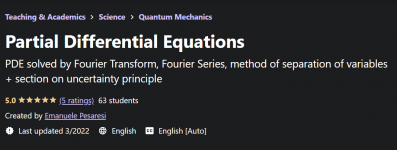#### Ruchika oberoi

Staff member### What you’ll learn​

• How to use the Fourier Trasforms to tackle the problem of solving PDE’s
• Fourier Transforms in one and multiple dimensions
• Method of separation of variables to solve the Heat equation (with exercises)
• Method of separation of variables to solve the Laplace equation in cartesian and polar coordinates (with exercises)
• How to apply the Fourier Transform to solve 2nd order ODE’s as well
• concept of streamlines
• Mathematical tricks
• How to derive Heisenberg Uncertainty Principle using concepts of Probability Theory

### Requirements​

• Calculus (especially: derivatives, integrals)
• Multivariable Calculus (especially: the Jacobian, the Laplacian, etc.)
• Complex Calculus (basics of Fourier series and residues could help)
• Some notions of probability theory (distributions, mean, variance)
• Complex numbers

### Who this course is for:​

• Students who are interested in Physics and in mathematical derivations of concepts